# 2-rankings of Graphs

Research Assistant at Miami University à Miami University
25 Sep 2015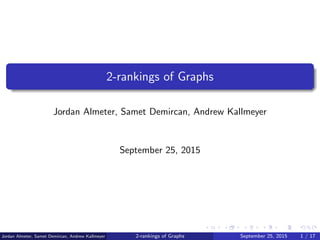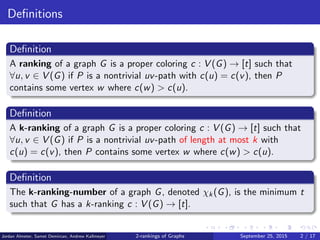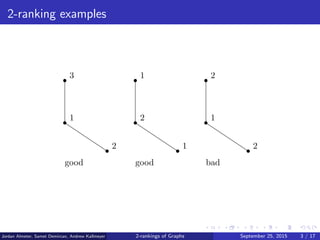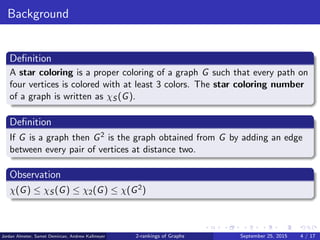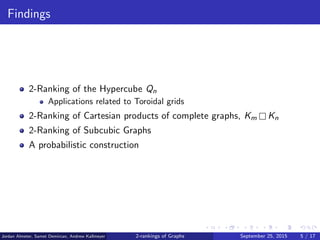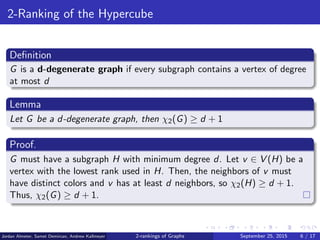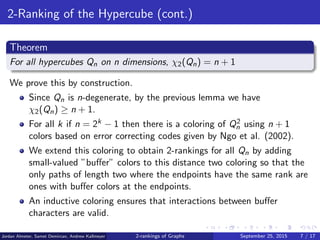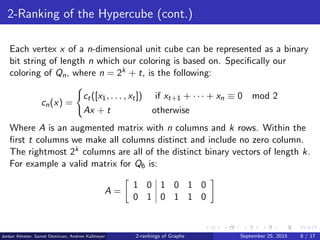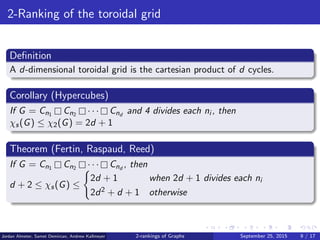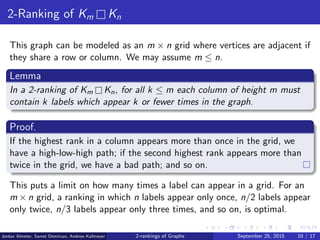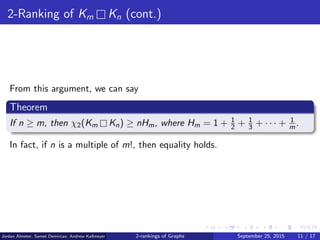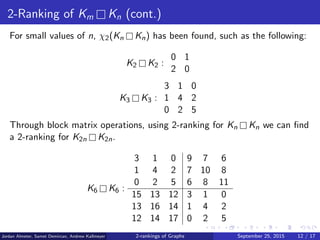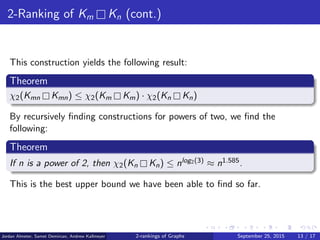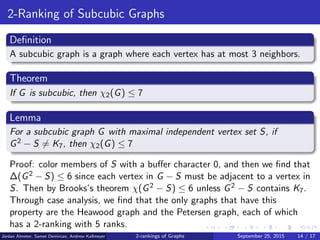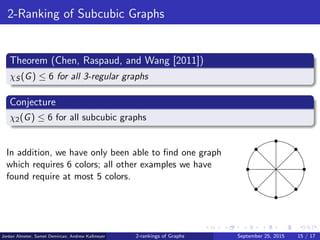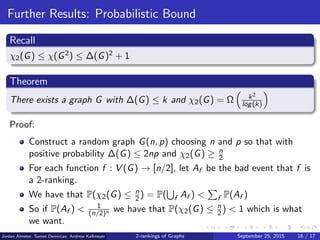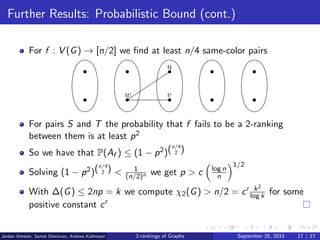1 sur 17

### 2-rankings of Graphs

• 1. 2-rankings of Graphs Jordan Almeter, Samet Demircan, Andrew Kallmeyer September 25, 2015 Jordan Almeter, Samet Demircan, Andrew Kallmeyer 2-rankings of Graphs September 25, 2015 1 / 17
• 2. Deﬁnitions Deﬁnition A ranking of a graph G is a proper coloring c : V (G) → [t] such that ∀u, v ∈ V (G) if P is a nontrivial uv-path with c(u) = c(v), then P contains some vertex w where c(w) > c(u). Deﬁnition A k-ranking of a graph G is a proper coloring c : V (G) → [t] such that ∀u, v ∈ V (G) if P is a nontrivial uv-path of length at most k with c(u) = c(v), then P contains some vertex w where c(w) > c(u). Deﬁnition The k-ranking-number of a graph G, denoted χk(G), is the minimum t such that G has a k-ranking c : V (G) → [t]. Jordan Almeter, Samet Demircan, Andrew Kallmeyer 2-rankings of Graphs September 25, 2015 2 / 17
• 3. 2-ranking examples 1 2 2 2 1 1 1 3 2 good good bad Jordan Almeter, Samet Demircan, Andrew Kallmeyer 2-rankings of Graphs September 25, 2015 3 / 17
• 4. Background Deﬁnition A star coloring is a proper coloring of a graph G such that every path on four vertices is colored with at least 3 colors. The star coloring number of a graph is written as χS (G). Deﬁnition If G is a graph then G2 is the graph obtained from G by adding an edge between every pair of vertices at distance two. Observation χ(G) ≤ χS (G) ≤ χ2(G) ≤ χ(G2) Jordan Almeter, Samet Demircan, Andrew Kallmeyer 2-rankings of Graphs September 25, 2015 4 / 17
• 5. Findings 2-Ranking of the Hypercube Qn Applications related to Toroidal grids 2-Ranking of Cartesian products of complete graphs, Km Kn 2-Ranking of Subcubic Graphs A probabilistic construction Jordan Almeter, Samet Demircan, Andrew Kallmeyer 2-rankings of Graphs September 25, 2015 5 / 17
• 6. 2-Ranking of the Hypercube Deﬁnition G is a d-degenerate graph if every subgraph contains a vertex of degree at most d Lemma Let G be a d-degenerate graph, then χ2(G) ≥ d + 1 Proof. G must have a subgraph H with minimum degree d. Let v ∈ V (H) be a vertex with the lowest rank used in H. Then, the neighbors of v must have distinct colors and v has at least d neighbors, so χ2(H) ≥ d + 1. Thus, χ2(G) ≥ d + 1. Jordan Almeter, Samet Demircan, Andrew Kallmeyer 2-rankings of Graphs September 25, 2015 6 / 17
• 7. 2-Ranking of the Hypercube (cont.) Theorem For all hypercubes Qn on n dimensions, χ2(Qn) = n + 1 We prove this by construction. Since Qn is n-degenerate, by the previous lemma we have χ2(Qn) ≥ n + 1. For all k if n = 2k − 1 then there is a coloring of Q2 n using n + 1 colors based on error correcting codes given by Ngo et al. (2002). We extend this coloring to obtain 2-rankings for all Qn by adding small-valued ”buﬀer” colors to this distance two coloring so that the only paths of length two where the endpoints have the same rank are ones with buﬀer colors at the endpoints. An inductive coloring ensures that interactions between buﬀer characters are valid. Jordan Almeter, Samet Demircan, Andrew Kallmeyer 2-rankings of Graphs September 25, 2015 7 / 17
• 8. 2-Ranking of the Hypercube (cont.) Each vertex x of a n-dimensional unit cube can be represented as a binary bit string of length n which our coloring is based on. Speciﬁcally our coloring of Qn, where n = 2k + t, is the following: cn(x) = ct([x1, . . . , xt]) if xt+1 + · · · + xn ≡ 0 mod 2 Ax + t otherwise Where A is an augmented matrix with n columns and k rows. Within the ﬁrst t columns we make all columns distinct and include no zero column. The rightmost 2k columns are all of the distinct binary vectors of length k. For example a valid matrix for Q6 is: A = 1 0 1 0 1 0 0 1 0 1 1 0 Jordan Almeter, Samet Demircan, Andrew Kallmeyer 2-rankings of Graphs September 25, 2015 8 / 17
• 9. 2-Ranking of the toroidal grid Deﬁnition A d-dimensional toroidal grid is the cartesian product of d cycles. Corollary (Hypercubes) If G = Cn1 Cn2 · · · Cnd and 4 divides each ni , then χs(G) ≤ χ2(G) = 2d + 1 Theorem (Fertin, Raspaud, Reed) If G = Cn1 Cn2 · · · Cnd , then d + 2 ≤ χs(G) ≤ 2d + 1 when 2d + 1 divides each ni 2d2 + d + 1 otherwise Jordan Almeter, Samet Demircan, Andrew Kallmeyer 2-rankings of Graphs September 25, 2015 9 / 17
• 10. 2-Ranking of Km Kn This graph can be modeled as an m × n grid where vertices are adjacent if they share a row or column. We may assume m ≤ n. Lemma In a 2-ranking of Km Kn, for all k ≤ m each column of height m must contain k labels which appear k or fewer times in the graph. Proof. If the highest rank in a column appears more than once in the grid, we have a high-low-high path; if the second highest rank appears more than twice in the grid, we have a bad path; and so on. This puts a limit on how many times a label can appear in a grid. For an m × n grid, a ranking in which n labels appear only once, n/2 labels appear only twice, n/3 labels appear only three times, and so on, is optimal. Jordan Almeter, Samet Demircan, Andrew Kallmeyer 2-rankings of Graphs September 25, 2015 10 / 17
• 11. 2-Ranking of Km Kn (cont.) From this argument, we can say Theorem If n ≥ m, then χ2(Km Kn) ≥ nHm, where Hm = 1 + 1 2 + 1 3 + · · · + 1 m . In fact, if n is a multiple of m!, then equality holds. Jordan Almeter, Samet Demircan, Andrew Kallmeyer 2-rankings of Graphs September 25, 2015 11 / 17
• 12. 2-Ranking of Km Kn (cont.) For small values of n, χ2(Kn Kn) has been found, such as the following: K2 K2 : 0 1 2 0 K3 K3 : 3 1 0 1 4 2 0 2 5 Through block matrix operations, using 2-ranking for Kn Kn we can ﬁnd a 2-ranking for K2n K2n. K6 K6 : 3 1 0 9 7 6 1 4 2 7 10 8 0 2 5 6 8 11 15 13 12 3 1 0 13 16 14 1 4 2 12 14 17 0 2 5 Jordan Almeter, Samet Demircan, Andrew Kallmeyer 2-rankings of Graphs September 25, 2015 12 / 17
• 13. 2-Ranking of Km Kn (cont.) This construction yields the following result: Theorem χ2(Kmn Kmn) ≤ χ2(Km Km) · χ2(Kn Kn) By recursively ﬁnding constructions for powers of two, we ﬁnd the following: Theorem If n is a power of 2, then χ2(Kn Kn) ≤ nlog2(3) ≈ n1.585. This is the best upper bound we have been able to ﬁnd so far. Jordan Almeter, Samet Demircan, Andrew Kallmeyer 2-rankings of Graphs September 25, 2015 13 / 17
• 14. 2-Ranking of Subcubic Graphs Deﬁnition A subcubic graph is a graph where each vertex has at most 3 neighbors. Theorem If G is subcubic, then χ2(G) ≤ 7 Lemma For a subcubic graph G with maximal independent vertex set S, if G2 − S = K7, then χ2(G) ≤ 7 Proof: color members of S with a buﬀer character 0, and then we ﬁnd that ∆(G2 − S) ≤ 6 since each vertex in G − S must be adjacent to a vertex in S. Then by Brooks’s theorem χ(G2 − S) ≤ 6 unless G2 − S contains K7. Through case analysis, we ﬁnd that the only graphs that have this property are the Heawood graph and the Petersen graph, each of which has a 2-ranking with 5 ranks. Jordan Almeter, Samet Demircan, Andrew Kallmeyer 2-rankings of Graphs September 25, 2015 14 / 17
• 15. 2-Ranking of Subcubic Graphs Theorem (Chen, Raspaud, and Wang ) χS (G) ≤ 6 for all 3-regular graphs Conjecture χ2(G) ≤ 6 for all subcubic graphs In addition, we have only been able to ﬁnd one graph which requires 6 colors; all other examples we have found require at most 5 colors. Jordan Almeter, Samet Demircan, Andrew Kallmeyer 2-rankings of Graphs September 25, 2015 15 / 17
• 16. Further Results: Probabilistic Bound Recall χ2(G) ≤ χ(G2) ≤ ∆(G)2 + 1 Theorem There exists a graph G with ∆(G) ≤ k and χ2(G) = Ω k2 log(k) Proof: Construct a random graph G(n, p) choosing n and p so that with positive probability ∆(G) ≤ 2np and χ2(G) ≥ n 2 For each function f : V (G) → [n/2], let Af be the bad event that f is a 2-ranking. We have that P(χ2(G) ≤ n 2 ) = P( f Af ) < f P(Af ) So if P(Af ) < 1 (n/2)n we have that P(χ2(G) ≤ n 2 ) < 1 which is what we want. Jordan Almeter, Samet Demircan, Andrew Kallmeyer 2-rankings of Graphs September 25, 2015 16 / 17
• 17. Further Results: Probabilistic Bound (cont.) For f : V (G) → [n/2] we ﬁnd at least n/4 same-color pairs u vw For pairs S and T the probability that f fails to be a 2-ranking between them is at least p2 So we have that P(Af ) ≤ (1 − p2)(n/4 2 ) Solving (1 − p2)(n/4 2 ) < 1 (n/2)n we get p > c log n n 1/2 With ∆(G) ≤ 2np = k we compute χ2(G) > n/2 = c k2 log k for some positive constant c Jordan Almeter, Samet Demircan, Andrew Kallmeyer 2-rankings of Graphs September 25, 2015 17 / 17